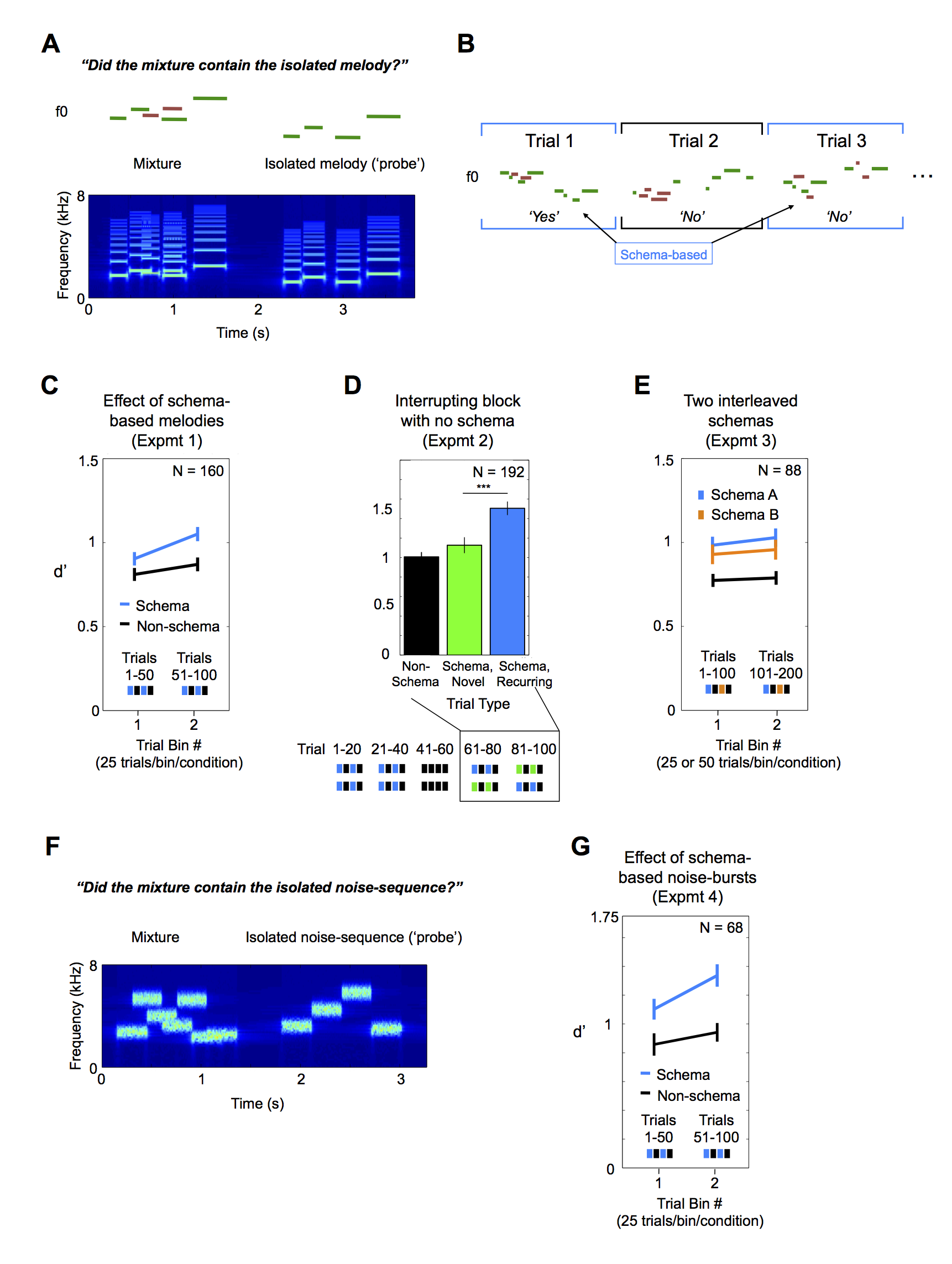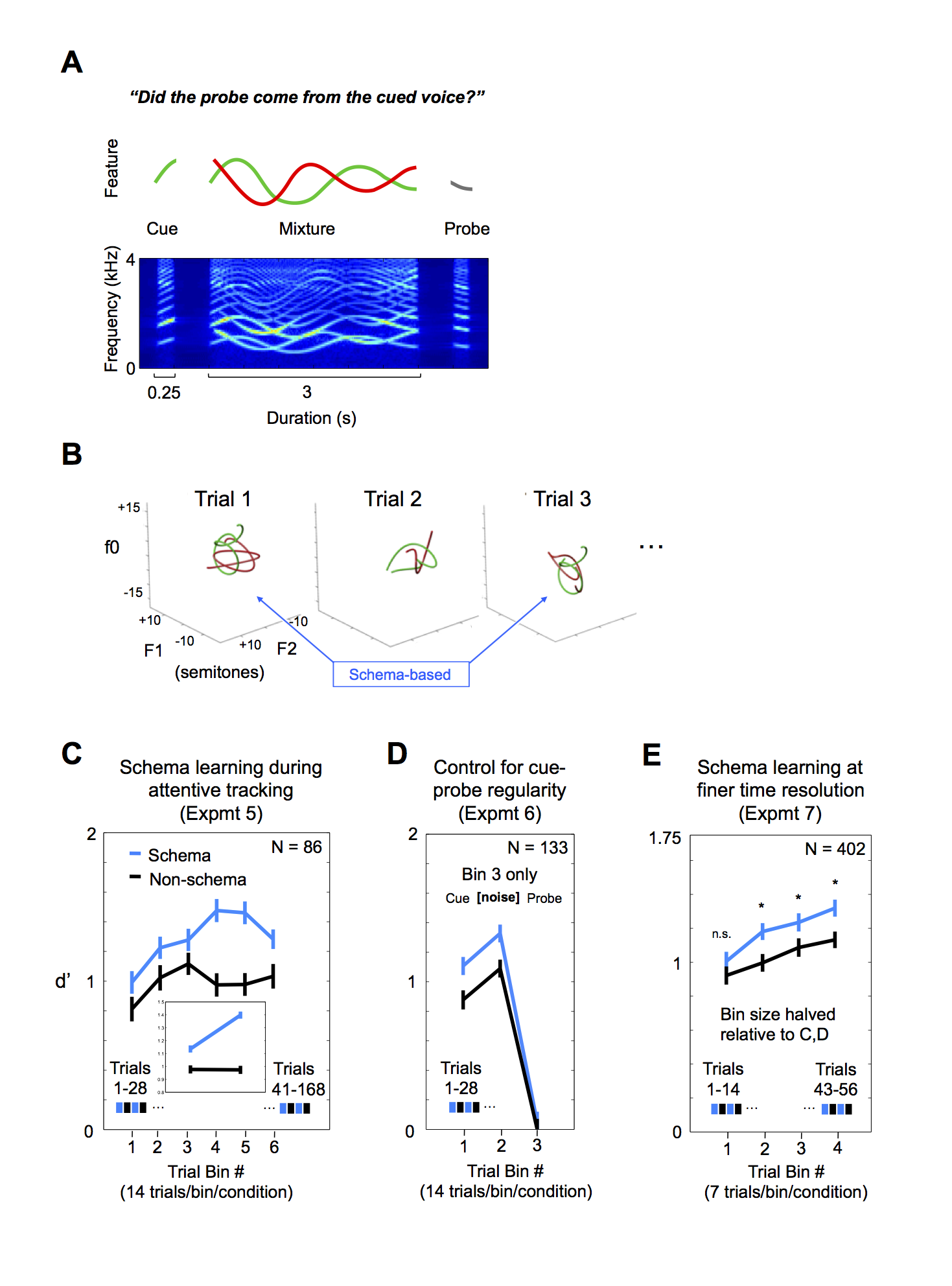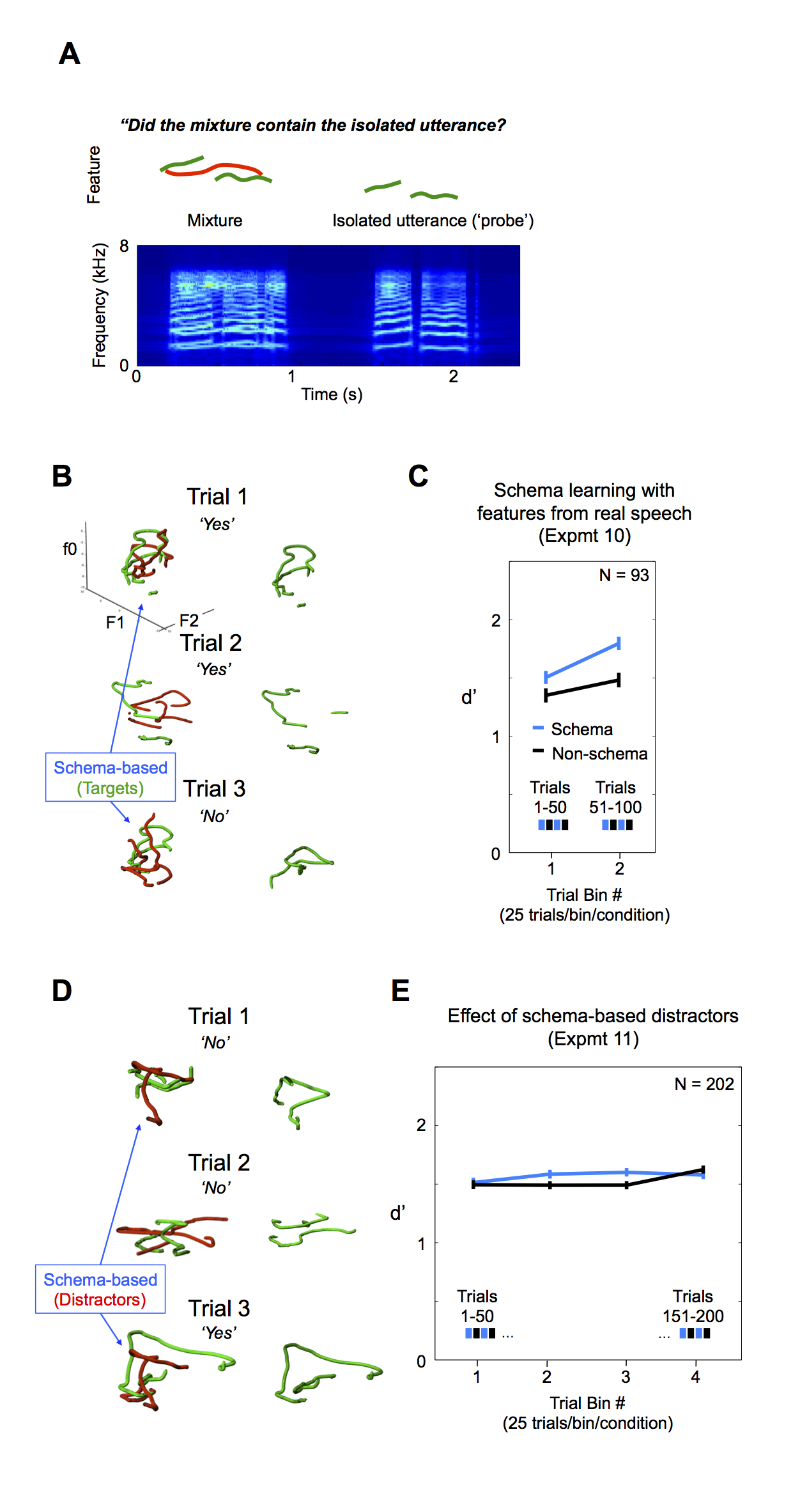Schema Learning for the Cocktail Party Problem

Kevin J.P. Woods and Josh H. McDermott

Proceedings of the National Academy of Sciences, vol.115 pp. E3313-E3322, Apr 2018.

Paradigm 1: Detection of discrete-tone melodiesSchema learning in melody segregation.
(A) Schematic of the trial structure (Upper) and a spectrogram of a sample stimulus (Lower). A target melody (green line segments) was presented concurrently with two distractor notes (red line segments), followed by a probe melody (green line segments). Listeners judged whether the probe melody matched the target melody in the mixture. The probe melody was transposed up or down in pitch by a random amount.
(B) Schematic of the basic experiment structure. On every other trial the target melody was generated from a common schema. On schema-based trials, the melody in the mixture was drawn from the schema 50% of the time, while the probe was always drawn from the schema.

Stimulus Examples: Melodies

(C) Results of experiment 1: recognition of melodies amid distractor tones with and without schemas (n = 160). Error bars throughout this figure denote the SEM. (D) Results of experiment 2: effect of an intervening trial block on learned schema (n = 192). Listeners were exposed to a schema, then completed a block without the schema, and then completed two additional blocks, one containing the original schema and one containing a new schema. The order of the two blocks was counterbalanced across participants. (Lower) The two rows of the schematic depict the two possible block orders. (Upper) The data plotted are from the last two blocks. (E) Results of experiment 3: effect of multiple interleaved schemas (n = 88). Results are plotted separately for the two schemas used for each participant, resulting in 25 and 50 trials per bin for the schema and non-schema conditions, respectively. (F) Spectrogram of a sample stimulus from experiment 4. Stimulus and task were analogous to those of experiment 1, except that noise bursts were used instead of tones.

Stimulus Examples: Noise-burst sequences

(G) Results of Exp.4: recognition of noise-burst sequences amid distractor bursts, with and without schemas (n = 68).

Answer key for example trials (melodies): Y,Y,N,Y,Y,Y

Answer key for example trials (noise bursts): Y

Paradigm 2: Attentive tracking of smooth pitch-formant contoursSchema learning in attentive tracking of synthetic voices.
(A) Schematic of the trial structure (Upper) and spectrogram of an example stimulus (Lower). A target voice (green curve) was presented concurrently with a distractor voice (red curve). Both voices varied smoothly but stochastically over time in three feature dimensions: f0, F1, and F2 (the fundamental frequency and first two formants; for clarity the schematic only shows variation in a single dimension). Voices in a mixture were constrained to cross at least once in each dimension. Listeners were cued beforehand with the initial portion of the target voice. Following the mixture, listeners were presented with a probe stimulus that was the ending portion of one of the voices and judged whether this probe came from the target.
(B) Schematic of the experiment structure. On every other trial the target voice was generated from a common schema. Voices are depicted in three dimensions. f0, F1, and F2 are plotted in semitones relative to 200, 500, and 1,500 Hz, respectively.

Stimulus Examples

(C) Results of experiment 5: effect of schemas on attentive tracking (n = 86). The Inset denotes results with trials binned into 42 trials per condition to maximize power for an interaction test (reported in text). Error bars throughout this figure denote the SEM.
(D) Results of experiment 6: a control experiment to ensure listeners could not perform the task with cues and probes alone (n = 146). In the last one-third of trials, the voice mixture was replaced with noise. (E) Schema learning on a finer time scale (n = 402). Data from the first 56 trials of experiments 5 and 6 were combined with new data from experiment 7 and replotted with seven trials per bin. The finer binning reveals similar performance at the outset of the experiment, as expected. n.s., not significant. *P < 0.05.

Answer key for example trials: Y,Y,N,Y,Y

Paradigm 3: Segregation of resynthesized speechSchema learning in segregation of resynthesized speech utterances.
(A) Schematic of the trial structure (Upper) and a spectrogram of an example stimulus from experiments 10 and 11 (Lower). A target utterance (green curve) was presented concurrently with a distractor utterance (red curve) and was followed by a probe utterance (second green curve). The utterances were synthesized from the pitch and formant contours of speech excerpts. For clarity the schematic only shows variation in a single dimension. Because only the first two formants were used, and because unvoiced speech segments were replaced with silence, the utterances were unintelligible. Listeners judged whether the probe utterance had also appeared in the mixture. When this was the case, the probe utterance was transposed in pitch and formants from the target utterance in the mixture and was time-dilated or compressed.
(B) Schematic of the structure of experiment 10. On every other trial the target utterance was generated from a common schema. Utterances are depicted in three dimensions.

Stimulus Examples

Schema-based targets (Expt.10)

(C) Results of experiment 10: effect of schemas on the segregation of speech-like utterances (n = 89). Error bars here and in E denote the SEM.

(D) Schematic of structure of experiment 11. On every other trial, the distractor utterance was generated from a common schema.

Schema-based distractors (Expt.11)

(E) Results of experiment 11 (n = 202).

Answer key for example trials (Exp10): Y,Y,N,Y,N

Answer key for example trials (Exp11): Y,Y,N,Y,Y